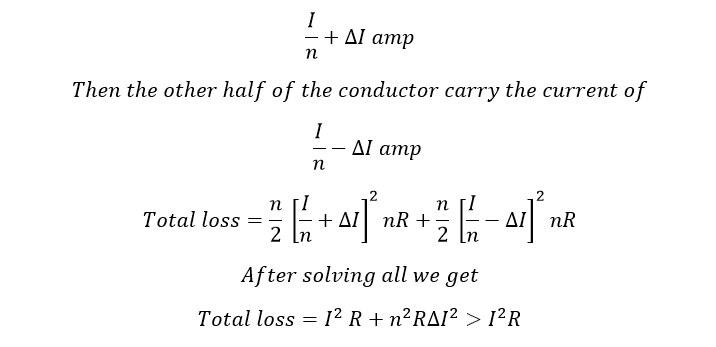# What is Skin Effect | Reduce Skin Effect in Transmission Lines

## What is Skin Effect:

The tendency of the alternating current to concentrate near the surface of a conductor is called skin effect. The direct current distribution in a conductor is uniform. But the alternating current distribution in a conductor is not uniform. The current density near the surface is more than near to the center of the conductor which means the current flow in the surface of the conductor is higher than the current flow in the center of the conductor. It is affected by the frequency of the current. If the frequency of the current is more the current distribution is more non-uniform. This effect is called as skin effect. The effective cross sectional area of the conductor through which current flows is reduce.

Let’s we take a conductor in solid state and bundled conductor.

In that R is the resistance of the solid conductor and I is the current through the conductor. N is the number of strands, nR is the resistance of each strand and the current through each strand is I/n..

Learn More:   What Is FTP (File Transfer Protocol) | SFTP | TFTP Full Explanation

Let assume half of the conductor n/2 carry the currents ofHere you can see that the effective resistance of alternating current is more than the direct current. oif the current distribution is not uniform.

At the same time, the inductance of each strand will be varied according to its position. The strand near the center are surrounded by a greater magnetic flux hence we get larger inductance. The flux linking the strand decreases as we move towards the outer strand. Therefore, the outside strand conduct more current than inside strand. Also the skin effect is purely depending upon the resistivity, frequency, conductor size and permeability of the conductor.

Note: The skin effect is negligible for the system frequency is less than 50 Hz and the conductor diameter is less than 1 cm.

### How to reduce the Skin Effect:

• ACSR bundled conductor is used to reduce the skin effect. ACSR conductors are the steel is placed inside of or center of the conductor and the aluminium conductor is positioned around steel wire. The steel increased the strength of the conductor but reduced the surface area of the conductor. Thus, the current flow mostly in the outer layer of the conductor and no current is carried in the centre of the conductor. Thus, reduced the proximity effect on the conductor.
• Use cable material with less magnetic permeability. (This reduces the effect, but usually comes at the cost of higher basic resistance per unit length, so it may be OK if the lines are short.)
• Reduce the size of the conductor.
• Increasing the voltage by reducing the current which decreases the skin effect in the same conductor.
Learn More:   Short Transmission Line Definition Equivalent circuit Phasor diagram Transmission Efficiency